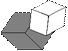Italian Journal of Pure and Applied Mathematics
ISSN 2239-0227Issue n. 43-2020

Click on the picture below to obtain the whole PDF:Click on the appropriate line below to get the corresponding section:

== It will be possible to download each single paper from this list before the end of March 2020 ==

1.     Upper class functions on a controlled contraction principle in partial $S$-metric spaces

2.     Characterization of some linear groups by their conjugacy class sizes

3.     On new class of contra continuity in nano topology

4.     Heptavalent symmetric graphs of order $8p 5. On almost generalized pseudo-Ricci symmetric spacetime 6. Some remarks on generalizations of prime submodules 7. A new view of closed-CS-module 8. Hesitant fuzzy sets approach to ideal theory in ordered$\Gamma$-semigroups 9. Intuitionistic fuzzy rare$\alpha$-continuity 10. En-semi prime subacts over monoids with zero 11. Join hesitant fuzzy filters of residuated lattices 12. Application of differential transformation method for solving prey predator model with holling type I 13. Some classes of mappings on generalized quaternion metric spaces II 14. On two-sided group digraphs and graphs 15. A characterization of simple group$S_4(7)$16. Redefined neutrosophic filters in BE-algebras 17. More on almost countably compact spaces 18. Fixed point results with$\Omega $-distance by utilizing simulation functions 19. On coefficient inequalities for certain subclasses of meromorphic bi-univalent functions 20. A note on unitarily invariant norm inequalities for accretive-dissipative operator matrices 21. Limiting direction of Julia sets and infinite radial order of solutions to complex linear differential equations 22. Intuitionistic fuzzy ideals on approximation systems 23. On modular flats and pushouts of matroids 24. Chebyshev wavelet method (CWM) for the numerical solutions of fractional boundary value problems 25. Fuzzy zero suffix algorithm to solve fully fuzzy transportation problems by using element-wise operations 26. Pricing European call options with default risk under a jump-diffusion model via FFT transform 27. Estimation for the parameter of a class of diffusion processes 28. Relationships between tropical eigenvectors and tropical fixed points of the group$GL(2,\mathbb{R})$29.$L^{\infty }$-asymptotic behavior for a finite element approximation to optimal control problems 30. Rings with strongly algebraically closed lattices 31. On the$n^*-$and$\gamma_n^*-$complete fuzzy hypergroups 32. Bifurcations of Liouville tori of generalized two-fixed center problem 33. Optimization technique for solving fuzzy partial differential equations under strongly generalized differentiability 34. On some properties of certain subclasses of univalent functions 35. On uniformly primary hyperideals and uniformly 2-absorbing primary hyperideals 36. Classes of weighted tent function spaces and mixed norms with some applications 37. Toward strictly singular fractional operator restricted by Fredholm-Volterra in Sobolev space 38. Nonlinear left$*$-Lie triple mappings of standard operator algebras 39. A common fixed point theorem without continuity under weak compatible mappings in uniform convex Banach spaces 40. A further study on the hyperideals of ordered semihypergroups 41. Finite group with coincide automizer and central automorphism of subgroups 42. Ricci semi-symmetric normal complex contact metric manifolds 43. The path graph of the amalgamated graph of C$_{3}$and C$_{n}$at an edge or at a vertex 44. A characterization of some alternating group by its order and special conjugacy class sizes 45. Invo-clean rings associated with central polynomials 46. On developing an optimal Jarratt-like class for solving nonlinear equations 47. Some spectral inclusion for strongly continuous semigroups operators 48. A reliable non-standard finite difference scheme for solving nonlinear biochemical reaction model 49. Numerical simulations for reactive nitrogen compounds pollution measurements in a stream using Saulyev method 50. Some results on$K$-frames 51. Analysis and implementation of kidney stones detection by applying segmentation techniques on computerized tomography scans 52. On conjugate trigonometrically$\rho$-convex functions 53. On decomposable$MS$-algebras 54. Common fuzzy fixed points of$\alpha$-fuzzy mappings 55. Characterizations of almost \textit{PP}-ring for three important classes of rings 56. Stability of fixed point sets of generalized multivalued$\alpha$-$\psi$contraction of Ciric-Berinde type 57. Modules closed full large extensions of cyclic submodules are summands 58. Some generalized forms of soft compactness and soft Lindel\"{o}fness via soft$\alpha$-open sets 59. Trust-based Testbed for P2P digital library 60. A new contraction and existence theorems on fuzzy metric space with a graph 61. Reliability bounds of dependent linear consecutive k-out-of- n:G systems 62. Predator-prey model of Holling-type II with harvesting and predator in disease 63. Bipolar complex fuzzy sets and their properties 64. The impact of organizational and human factors on the successful implementation of ERP system in water authority of Jordan 65. Second type nabla Hukuhara differentiability for fuzzy functions on time scales 66. Characterization of generalized projective and injective soft modules 67. Fixed point theorem for contraction mappings in probabilistic normed spaces 68. Criteria and geometric properties for bounded univalent functions in the unit disk 69. Studying the solutions of the delay Sturm Liouville problems 70. Common fixed point of faintly compatible in fuzzy metric space 71. Operator inequalities involving improved Young inequality 72. On minimal$\lambda_{rc}$-open sets 73. Pseudo analytic approach to estimate drug transport and release in the annular section of human limbs 74. Some cryptographic properties of near bent functions over finite fields 75. On$(m,n)$-fully stable Banach algebra modules 76. Vertex {$(n,k)\$}-choosability of graphs

77.  Rough approximate operators based on fuzzy soft relation

78.  New classes of uniformly convex functions of fractional power on Banach space

79.  Asymptotic stability analysis of nonlinear systems with impulsive effects and disturbance input

80.  Numerical simulation of nonlinear fractional integrodifferential equations of Volterra type via power series expansion

81.  Qualitative behavior of a SIRS epidemic model with vaccination on heterogeneous networks

82.  The non-zero divisor graph of a ring